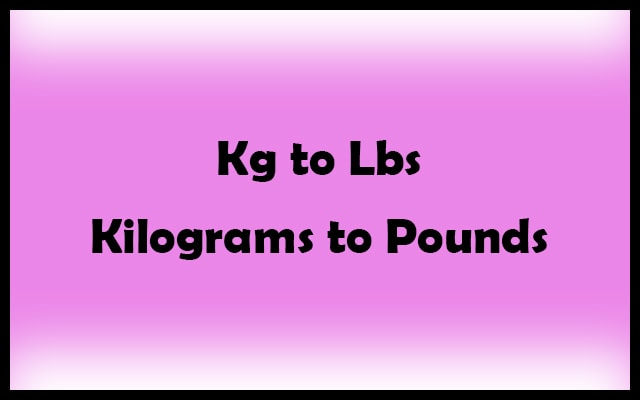# kg to lbs Calculator – Convert Kilograms to Pounds

Kilogram and Pound are the most used units for measuring weight. However, Kilogram (KG) is the International Standard unit for measuring mass. Then also surprisingly we have to use both of them. In the same way, we have to convert kg to lbs. In conclusion, the conversion of Kilograms to Pounds or vice versa will satisfy every need.

Kilograms (kg)

Pounds (lbs)

Related

The kg to lbs calculator is a tool that will convert Kg to Pounds in seconds. In addition, you can also do the reverse which is Pounds to kilograms. Here we have also discussed the mathematical process of converting Kilograms to Pounds. In the same fashion with this tool, you can also do the reverse conversion.#### Kilogram (kg)

Previously a Kilogram was the mass of 1000 cm3 of water. This definition is correct only if the water is pure. Presently the definition of a Kilogram is actually the mass of an International prototype consisting of Platinum-Iridium. This prototype is kept in the International Bureau of Weights and Measures.

Now presently it is used in almost all countries as the standard unit for mass. A new definition of Kilogram has been introduced at this instant in the year 2019. This latest definition has something related to Planck’s constant.

#### Pounds (lbs)

If you are from the United States of America or the United Kingdom then you must have used Pound more than Kilogram for determining weights. Kilogram in spite of being the standard unit is not accepted everywhere. As a result, we use tools like Kg to Pounds converter.

The concept of a pound was from the Roman Libra. Consequently, we use lb as a symbol for the pound. Like Kilogram has a prototype similarly Pound also has a prototype.

#### KILOGRAM VS POUND

This is the comparison between Kilogram (Kg) and Pound (lb). Let’s compare both of them. Also in like manner, we will know the relation between them.

1 kg = 2.2046226218 lbs
1 lbs = 0.45359237 kg

Above is the actual conversion rate of Kilograms to Pounds and vice versa. Using this data you can carry on any conversion. For example, convert 5 kg to lbs. Since we know that 1 kg = 2.2046226218 lbs therefore 5 kg = (5 X 2.2046226218) lbs. Hence, the final answer is 5 kg = 11.023113109 lbs.

## Why use kg to lbs calculator?

We know how to convert Kg to Pounds then why should we go for this tool? This section will clear all your doubts like this. In short, it is about why manual calculations are not recommended. Read these things to know the reasons.

1. As you know that the calculation of kg to lbs is easy but also complex. Just because the decimal multiplication consumes time. Remember it consumes time when you go for manual calculations. Do it in seconds by using our tool.
2. Since the calculation is complex. Therefore, the probability of getting the wrong answer is very much when you go for manual calculations. Get the most accurate result if you use our tool. This is possible only because the tool is pre-programmed. It uses its algorithms to give output. As a result, you can never expect the wrong answer.
3. Availability is another key factor behind using it. In today’s time every moment, you have mobile phones with you. Whereas on the other hand, the pen-paper may not be present. So, that is the reason why using tools are more recommended.

### How to use the Kilograms to Pound Converter tool?

Firstly, this tool will work only if you have an Internet connection. So make sure you have an Internet connection. Secondly, a device that can access the Internet. The user interface is very simple. You don’t need to be a Computer Science Engineer for using it. Anyone with very basic computer-using knowledge can easily operate this tool.

In your device (Mobile / Tablet / Laptop / Desktop) open a web browser and visit this website. When the website fully loads you are ready to go.

There is an input box where you need to enter the Kilogram. After that press the “Convert” button. It will display the results after converting kg to lbs. The result (Pounds) will appear at the bottom along with a calculation. Lastly, for a new conversions just click the reset button.

#### Kg to Pounds conversion table

Kilograms (kg) Pounds (lb)
0 kg 0 lb
0.1 kg 0.220 lb
1 kg 2.205 lb
2 kg 4.409 lb
3 kg 6.614 lb
4 kg 8.818 lb
5 kg 11.023 lb
6 kg 13.228 lb
7 kg 15.432 lb
8 kg 17.637 lb
9 kg 19.842 lb
10 kg 22.046 lb
20 kg 44.092 lb
30 kg 66.139 lb
40 kg 88.185 lb
50 kg 110.231 lb
60 kg 132.277 lb
70 kg 154.324 lb
80 kg 176.370 lb
90 kg 198.416 lb
100 kg 220.462 lb
1000 kg 2204.623 lb